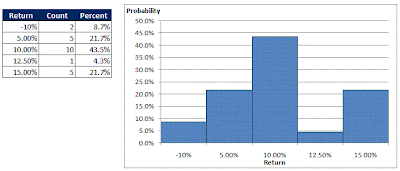## Tuesday, June 30, 2009

### Time Series Analysis: Univariate and Multivariate Distributions

Introductory Concepts in Time Series Analysis
A time series refers to a set of data that is ordered by time. Price, volume and return data are three examples of time series. Time series can be discrete or continuous. In a discrete series, the time interval between each observation is an integer value. Continuous series are ordered by real numbers, the entire set of numbers that form the number line and need not necessarily refer to integers.In the example above, we let x refer to the entire time series of stock prices and x can have many different values for its subscript i to refer to each individual observation in the time series. In this case, x0 refers to the price today of \$1.01. A single price is an observation. Strictly speaking, only xi before x0 are the realized observations, i.e. the historic prices.

Univariate and Multivariate Distributions and Time Series
When analyzing financial data, we often make comparisons involving more than one variable across time. When trading stocks, we may consider key information such as historical return, volume, dividend payout, profitability, sales growth etc. When a stock shows positive characteristics such as a low price-earnings ratio or strong historical price growth on high volume, we are more likely than not to expect positive future returns and buy into the stock.

Using terms borrowed from statistics and probability, we might be unknowningly making the assumption that the probability of a stock having a positive future return is greater than 50% given that its historical returns is more than 10% a year and its current profit growth is more than 20% annually. In mathematical notation, we may be assuming the following:

P[Future Return>0%|(Historical Return>10%, Current Profit Growth>20%)]>50%

If we are indeed making such assumptions, we must then believe that the future return of a stock given other information may take on multiple values with different probabilities. E.g.

P(Future Return = 5%|certain information=x) = 30%
P(Future Return = 10%|certain information=x)=15%
....

, where we let certain information and x be variables we use in our analysis such as profit growth =x% or volume =x lots traded etc.

The above are known as conditional probabilities. If we know the probability of observing certain information = x, P(certain information=x) = y%, we can convert these probabilities to joint probabilities using Bayes' rule.

P(A and B) = P (A|B) x P(B)

If we were to download 100 years of data for the Dow Jones Industrial Average and calculate the monthly return, we might observe the following univariate distribution.On the other hand, we can calculate the returns in the past one month and in the next one month for every month of data we have to obtain the following possible distribution, which looks like a cone. Basically, it assigns a certain probability to every possible combination of current and future returns.Thus it becomes apparent that by analyzing a set of variables to predict future return, we are inherently making probabilistic assumptions of future returns against available information. As such, we should shift our perspective of statistical distributions from a purely univariate case to a multivariate situation when studying related financial data.

The univarate case applies when the data we study has no relationship with each other. In the example above, the univariate distribution of monthly returns will be sufficient to tell us how much return we can expect in any month if we believe that historical returns do not affect future returns. If historical return share no relationship with future return, the probability of future return being a certain value x% will be the same regardless of historical return. If that is really the case, the multivariate distribution will be misleading. Hence, your chosen variables will determine your probability distribution which in turn affects your decision making process.

In reality, multivariate time series occur when we observe multiple related variables at the same point in time. An example will be price and volume. At time, t=0, you observe both the particular price and volume traded – 2 variables at the same time. If you assume that your series can go no more detailed than one day at a time, you can grudgingly argue that the open, high, low and closing prices are four variables observed at the same time. The crux of the issue is whether you correctly choose to include or exclude multiple variables in your analysis, to record an appropriate time series.

The distinction between these two time series is not trivial. Suppose you have been observing daily prices for a long time and you know that observing a price of \$1.00 today gives you a 5% chance of observing a price of \$1.20 tomorrow. Another person who observes both price and volume, might give only a 3% chance of observing a price of \$1.20 tomorrow if today’s volume is 1000 shares traded.

Parametric Distributions
In practice, tabulating raw data might yield clumsy distributions that are not useful such as in the chart below.In the case above, you will have dififculty inferring the probability of having a return of less than -10% or 14% or more than 15%. This happens when you have insufficient data or data that is not representative of what you believe to be the true distribution. To handle such situations, practitioners either apply smoothing techniques to their data or assume a parametic distribution such as the normal distribution or the student t distribution in their analysis.

Like what you have just read? Digg it or Tip'd it.
The objective of Finance4Traders is to help traders get started by bringing them unbiased research and ideas. Since late 2005, I have been developing trading strategies on a personal basis. Not all of these models are suitable for me, but other investors or traders might find them useful. After all, people have different investment/trading goals and habits. Thus, Finance4Traders becomes a convenient platform to disseminate my work...(Read more about Finance4Traders)